In Online Education NCERT Solutions for Class 8 Maths Chapter 12 Exponents and Powers Ex 12.1 are part of NCERT Solutions for Class 8 Maths. Here we have given NCERT Solutions for Class 8 Maths Chapter 12 Exponents and Powers Ex 12.1.

 Board CBSE Textbook NCERT Class Class 8 Subject Maths Chapter Chapter 12 Chapter Name Exponents and Powers Exercise Ex 12.1 Number of Questions Solved 7 Category NCERT Solutions

## Online Education NCERT Solutions for Class 8 Maths Chapter 12 Exponents and Powers Ex 12.1

Question 1.
Evaluate :
(i) $${ 3 }^{ -2 }$$
(ii) $${ -4 }^{ -2 }$$
(iii) $$({ \frac { 1 }{ 2 } ) }^{ -5 }$$
Solution.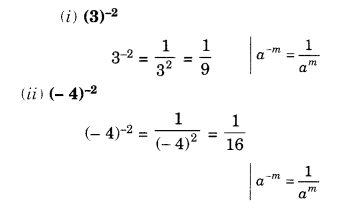Question 2.
Simplify and express the result in power notation with positive exponent.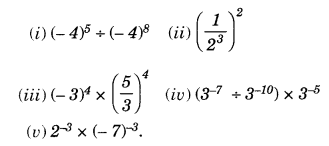Solution.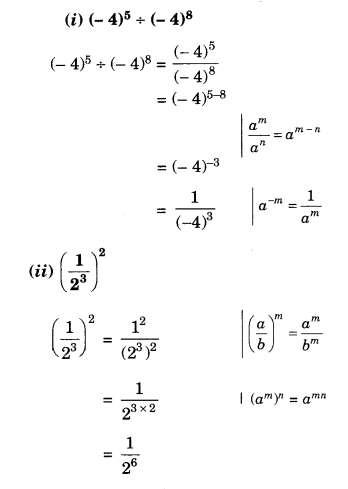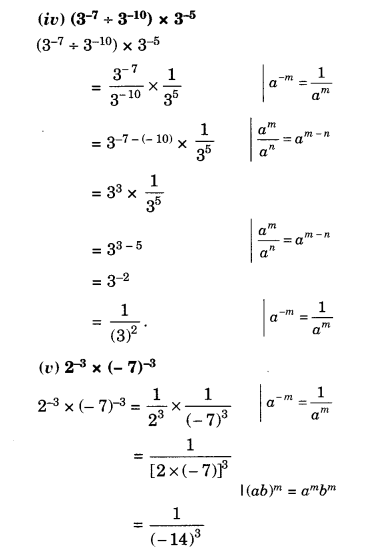Question 3.
Fmd the value of:Solution.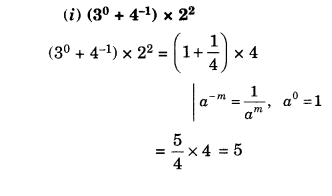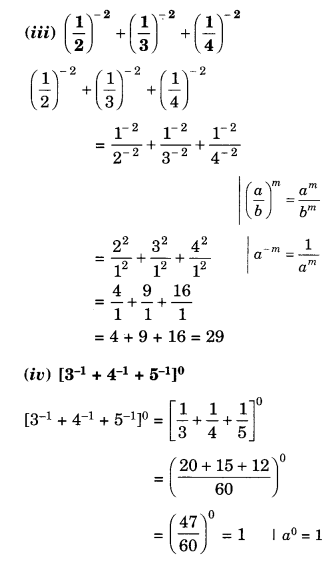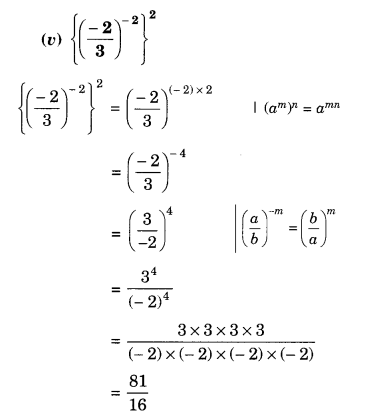Question 4.
Evaluate
(i) $$\frac { { 8 }^{ -1 }\times { 5 }^{ 3 } }{ { 2 }^{ -4 } }$$
(ii) $$({ 5 }^{ -1 }\times { 2 }^{ -1 })\times { 6 }^{ -1 }$$
Solution.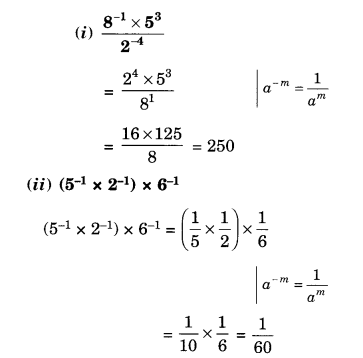Question 5.
Find the value of m for which $${ 5 }^{ m }+{ 5 }^{ -3 }={ 5 }^{ 5 }$$
Solution.Question 6.
Evaluate :Solution.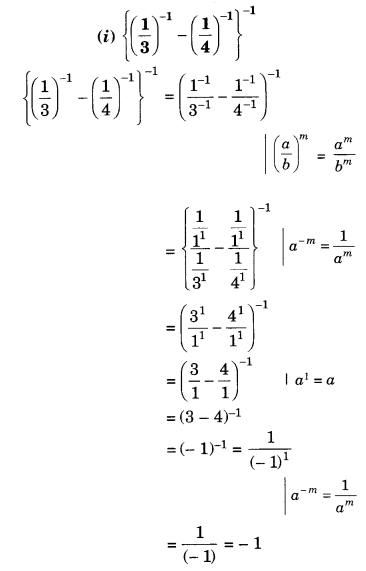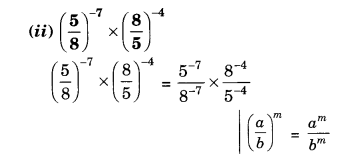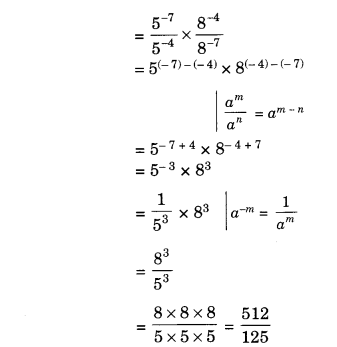Question 7.
Simplify:
(i) $$\frac { 25\times { t }^{ -4 } }{ { 5 }^{ -3 }\times 10\times { t }^{ -8 } }$$ (t ≠ 0)
(ii) $$\frac { { 3 }^{ -5 }\times { 10 }^{ -5 }\times 125 }{ { 5 }^{ -7 }\times { 6 }^{ -5 } }$$
Solution.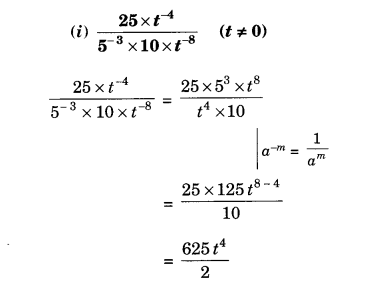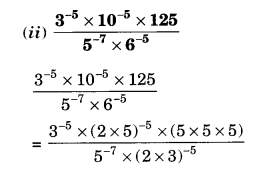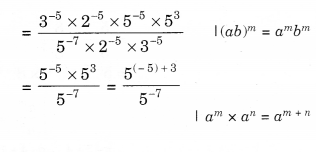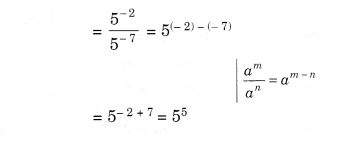We hope the NCERT Solutions for Class 8 Maths Chapter 12 Exponents and Powers Ex 12.1 help you. If you have any query regarding NCERT Solutions for Class 8 Maths Chapter 12 Exponents and Powers Ex 12.1, drop a comment below and we will get back to you at the earliest.